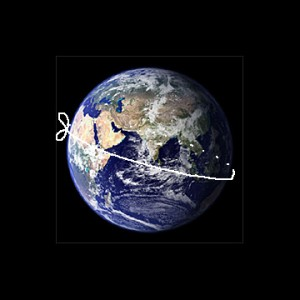## Algebra - A fascinating subject

Algebra is when most children get introduced to the concepts of “abstract” mathematics. Suddenly their world gets involved in quantities like “x” and “y”s. And if not introduced properly it can lead to fear of the subject.  But if taught properly they can see that the “x” and “y” are like words that form a beautiful poem and they’ll delight themselves when they learn this secret language and understand the beauty of algebra.

Understanding and practice can go a long way in helping children here. Sites like EduGain (www.edugain.com) can help children in doing this.

Suppose you take a long, really long string. You then cut out enough of it so that it can go all the way around the earth (I told you it was really long).So now you have this string that’s on the ground and encircling the earth along the equator.Now suppose you add another 30 centimeters to that string (that’s about the length of your long ruler). We then smoothen it out by pulling it up from the ground all over the equator so that it forms a proper circle again.

The question is “How high do you think the string will be above the ground now?”

Sounds very hard to solve, right. But let’s see…

We know that the circumference of a circle is C = 2 x ? x R, where R is the radius.
(don’t worry about ? – let’s just use the value of “3? for now)

So, C = 2 x 3 x R = 6 x R

Now we don’t know the exact radius of the earth here, but we don’t need to. Let’s leave it as a symbol R. So the length of the original string = 6 x R. We’ll just call it 6R (and know it means the value of R, whatever it is, multiplied by 6).

So C = 6R

Now we added 30 cm to C. The length of the new string is now C+30.

What we need to find out is how much R increases.

Let’s say that R increased by some length Z. The value of Z is what we want to find

So for the string, we can say it has a new circumference (Cnew) a new radius (Rnew)

We also know that since it’s a circle

Cnew = 6Rnew

And we know that the new circumference Cnew = C+30,
and that
Rnew = R + Z (remember we already assumed R increased by a length Z which we are trying to find out).

So we get

C + 30 = 6(R + Z).

Expanding this, we get,

C + 30 = 6R + 6Z

But we already know that 6R = C

Let’s replace the 6R by C on the right side

C + 30 = C + 6Z

The two “C”s cancel out, leaving us with

30 = 6Z, or

Z = 30/6 = 5

Amazing isn’t it. The new string would be 5 cm above the ground all everywhere. Just by adding 30 cm extra.

And what’s more amazing – we solved it through Algebra